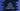# Java program to check if a number is a happy number or not## Java program to check if a number is a happy number or not:

In this post, we will learn how to check if a number is a happy number or not. This program will take one number as input from the user and print one message if it is a happy number or not.

### Happy number:

A number is called a happy number if we get 1 when we find the sum of square of digits of the number repeatedly till we get a one digit number. For example, 28 is a happy number because,

``````28 = 2^2 + 8^2 = 4 + 64 = 68
68 = 6^2 + 8^2 = 36 + 64 = 100
100 = 1^2 + 0^2 + 0^2 = 1 + 0 + 0 = 1``````

One interesting point is that the result for sum of digits of a number for a unhappy number is always 4.

So, we have to keep finding the sum of square of digits of a number repeatedly until it becomes 1 or 4.

### Algorithm:

We will use the below algorithm to check for a happy number:

• Get the number from the user.
• Use a loop. This loop will run until the value of the number is 1 or 4. Inside this loop:

• Find the sum of squares of each digits of the number.
• Assign the sum value to the number variable.
• This loop will stop once the number become 1 or 4.
• If the current value of the number is 1, return true i.e. it is a happy number. Else, return false.

## Java program:

Let’s take a look at the below program:

``````import java.util.Scanner;

class Main {
static int findSumDigitSquare(int n) {
int sum = 0;
int lastDigit;
while (n > 0) {
lastDigit = n % 10;
sum += lastDigit * lastDigit;
n /= 10;
}
return sum;
}

static boolean isHappy(int n) {
if (n <= 0) {
return false;
}
while (!(n == 1 || n == 4)) {
n = findSumDigitSquare(n);
}

return n == 1;
}

public static void main(String[] args) {
Scanner sc = new Scanner(System.in);
int n;
System.out.println("Enter a number: ");
n = sc.nextInt();

if (isHappy(n)) {
System.out.println(n + " is a happy number");
} else {
System.out.println(n + " is not a happy number");
}
}
}``````

Here,

• isHappy method is used to check if a number is a happy number or not. It returns one boolean value, true if it is a happy number and false otherwise.

• If the provided number is less than or equal to 0, it returns false.
• The while loop will run continuously until the value of n becomes equal to 1 or 4. Inside the loop, we are finding the sum of squares of all the digits of the number and assigning that value to n.
• isHappy returns true only if the final value of n is 1, i.e. it is a happy number.
• findSumDigitSquare method is used to find the sum of square values of each digits of a number.

• It takes a number as the parameter and returns the final sum value.
• It initializes a variable sum as 0 to hold the sum of squares of each digit of the given number.
• The lastDigit variable is initialized to hold the last digit of the number temporarily.
• The while loop runs until the value of n is greater than 0.
• Inside the loop, it finds the last digit of the number, finds the square value of the last digit and adds it to the sum variable and removes the last digit of the number by dividing it by 10.
• This method returns the final sum.
• Inside main, we are reading a number entered by the user by using a Scanner object.
• The if-else condition uses isHappy method to check if the entered number is a happy number or not and it prints one message based on that.

### Output:

If you run the above program, it will print output as like below:

``````Enter a number:
123
123 is not a happy number

Enter a number:
97
97 is a happy number``````